### Home > GC > Chapter 7 > Lesson 7.1.2 > Problem7-16

7-16.
1. the geometric relationships in each of the diagrams below. For each one, write and solve an equation to find the value of the variable. Name any geometric property or conjecture that you used. Homework Help ✎

1.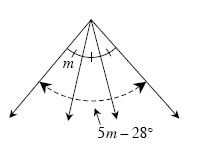2.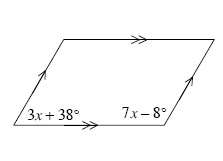3. ΔABC below is equilateral.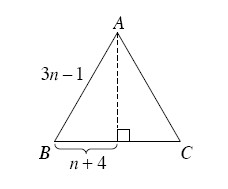4. C is the center of the circle below AB = 11x − 1 and CD = 3x + 12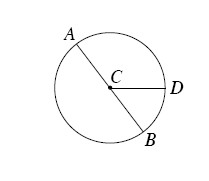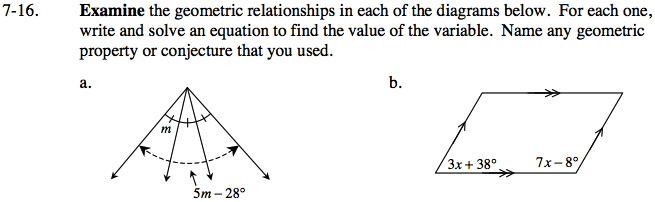How do the different measures of the angle relate to each other? How can you use this to solve for m?

m = 14°

3x + 38° + 7x - 8° = 180°

x = 15°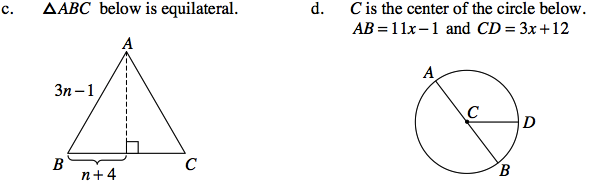How do each of the sides of the triangle relate to each other if the triangle is equilateral?